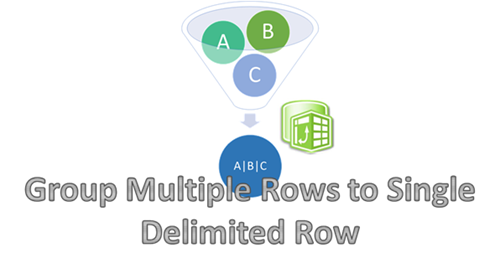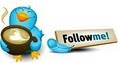#PowerPivot Concatinate vs Breakout• ### Question

•I am building a PowerPivot of orders that is built out of records of items on each order.

Example:

Order, Item, Cost, Quantity,Total

1234, Handle Bars, \$15, 1,\$15

1234, Seat, \$7,1,\$7

1234, Wheels, \$15,2,\$30

9874, Helmet, \$30,1,\$30

I need to be able to show the report grouped by order number with the Item descriptions concatinated into a singular field and the total number of items in the order, order total, and the Rank of the order with the largest total being #1.

Example:

Order, Item, Quantity,Total, Rank

1234, Handle Bars- Seat- Wheels- Helmet, 4, \$52, 1

9874, Helmet, 1, \$30, 2

And I also need to be able to show the item breakout by Rank.

Order, Item, Cost, Quantity,Total, Rank

1234, Handle Bars, \$15, 1,\$15,1

1234, Seat, \$7,1,\$7, 1

1234, Wheels, \$15,2,\$30, 1

9874, Helmet, \$30,1,\$30, 2

Any ideas?

Friday, January 4, 2013 10:05 PM

•Ok, I am a little short of time, so will post the solution (and explain it sometime later maybe)

1) Create a hidden calculated column called Rnk

`=RANKX(filter(ALL(Table1), [Order]=EARLIER([Order])), [Item],,1,DENSE)`

2) Create a hidden calculated column called ParRnk

`=if(Table1[Rnk]<>1, Table1[Rnk]-1)`

3) Create a hidden calculated column called ParItem

`=calculate(FIRSTNONBLANK(Table1[Item],1), FILTER(Table1, Table1[Rnk]=EARLIER(Table1[ParRnk])),VALUES(Table1[Order]))`

4) Create a calculated column called ConCol. This will give you the Item descriptions concatenated into a singular field

`=calculate(path(Table1[Item], Table1[ParItem]), CALCULATETABLE(filter(Table1, Table1[Rnk]=max(Table1[Rnk])),ALLEXCEPT(Table1,Table1[Order]))`

5) Create a measure called TotRank. This will give you Rank of the order with the largest total being #1.

`TotRank:=RANKX(All(Table1),CALCULATE(sum(Table1[Total]), ALLEXCEPT(Table1, Table1[ConCol], Table1[Order])),,,DENSE)`UpdateCheers,
Jason | www.SqlJason.comSaturday, January 5, 2013 5:34 PM

### All replies

•Ok, I am a little short of time, so will post the solution (and explain it sometime later maybe)

1) Create a hidden calculated column called Rnk

`=RANKX(filter(ALL(Table1), [Order]=EARLIER([Order])), [Item],,1,DENSE)`

2) Create a hidden calculated column called ParRnk

`=if(Table1[Rnk]<>1, Table1[Rnk]-1)`

3) Create a hidden calculated column called ParItem

`=calculate(FIRSTNONBLANK(Table1[Item],1), FILTER(Table1, Table1[Rnk]=EARLIER(Table1[ParRnk])),VALUES(Table1[Order]))`

4) Create a calculated column called ConCol. This will give you the Item descriptions concatenated into a singular field

`=calculate(path(Table1[Item], Table1[ParItem]), CALCULATETABLE(filter(Table1, Table1[Rnk]=max(Table1[Rnk])),ALLEXCEPT(Table1,Table1[Order]))`

5) Create a measure called TotRank. This will give you Rank of the order with the largest total being #1.

`TotRank:=RANKX(All(Table1),CALCULATE(sum(Table1[Total]), ALLEXCEPT(Table1, Table1[ConCol], Table1[Order])),,,DENSE)`UpdateCheers,
Jason | www.SqlJason.com•